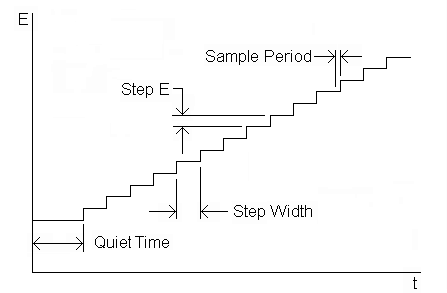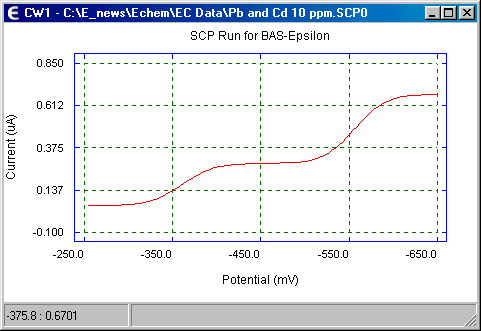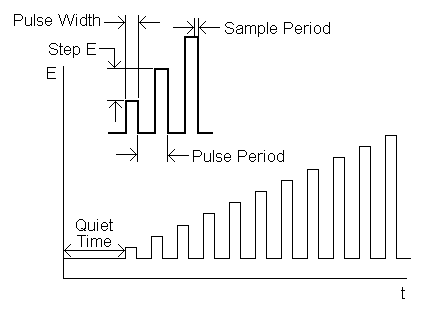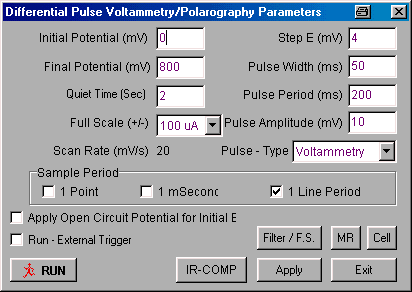# Pulse Voltammetric Techniques

#### Introduction

The basis of all pulse techniques is the difference in the rate of the decay of the charging and the faradaic currents following a potential step (or "pulse"). The charging current decays exponentially, whereas the faradaic current (for a diffusion-controlled current) decays as a function of 1/(time)½; that is, the rate of decay of the charging current is considerably faster than the decay of the faradaic current. The charging current is negligible at a time of 5RuCdl after the potential step (RuCdl is the time constant for the electrochemical cell, and ranges from µs to ms). Therefore, after this time, the measured current consists solely of the faradaic current; that is, measuring the current at the end of a potential pulse allows discrimination between the faradaic and charging currents.

The important parameters for pulse techniques are as follows:

1. Pulse amplitude is the height of the potential pulse. This may or may not be constant depending upon the technique.
2. Pulse width is the duration of the potential pulse.
3. Sample period is the time at the end of the pulse during which the current is measured.
4. For some pulse techniques, the pulse period or drop time must also be specified. This parameter defines the time required for one potential cycle, and is particularly significant for polarography (i.e., pulse experiments using a mercury drop electrode), where this time corresponds to the lifetime of each drop (i.e., a new drop is dispensed at the start of the drop time, and is knocked off once the current has been measured at the end of the drop time - note that the end of the drop time coincides with the end of the pulse width).

A number of different pulse techniques are available on the epsilon, which differ in their potential pulse wave forms, the number of sampling points, and whether a solid electrode (voltammetry) or a mercury drop electrode (polarography) is used. These are listed below. The discrimination against the charging current that is inherent in these techniques leads to lower detection limits (when compared to linear sweep techniques), which makes these techniques suitable for quantitative analysis.

## Sampled Current Polarography

Sampled current polarograph (SCP) is a modification of the classical DC polarography experiment, and was designed to reduce the effect of the changing surface area of the mercury drop electrode. The potential wave form is shown in Fig1 and the Change Parameters dialog box is shown in Fig2. The potential is varied in a series of steps, with the current sampled at the end of each step.Figure 1. Potential wave form for sampled current polarography.Figure 2. Change Parameters dialog box for sampled current polarography.

1. All potential values are entered in mV, and the Step Width is entered in ms.
2. If the Apply Open Circuit Potential for Initial E box is checked, then the open circuit potential will automatically be measured and used as the Initial Potential.
3. If the Run - External Trigger box is checked, the experiment is started from an external device using the Start In back-panel connection.
4. The Pulse Type must be specified when using a mercury electrode (CGME SMDE Mode selected in the Cell Stand dialog box). If Voltammetry is selected, the whole experiment is performed on a single mercury drop (after the Pre Run Drops); if Polarography is selected, a new drop is used for each data point.
5. The change in the applied potential for each step is defined by Step E.
6. The Scan Rate cannot be directly changed by the user, and is determined by Step E x 1/Sample Width.
7. Three options are available for the Sample Period:
• The current is measured once at the end of the Step Width (1 Point)
• The current is measured multiple times in 1 ms at the end of the Step Width, and averaged (1 mSecond)
• The current is measured multiple times over 1 line cycle at the end of the Step Width, and averaged (1 Line Period). The time required for 1 line cycle is the reciprocal of the line frequency (16.7 ms for 60 Hz, and 20 ms for 50 Hz). The line frequency is selected in the Setup / Manual Settings (I/O) dialog box.
Generally speaking, increasing the Sample Period increases the signal-to-noise ratio. However, the 1 Line Period option may not be possible for short Step Width values.
8. If the Apply Open Circuit Potential for Initial E box is checked, then the open circuit potential will automatically be measured and used as the Initial Potential.
9. When the experiment is started, the cell is held at the Initial Potential for the number of seconds defined by the Quiet Time.
10. There are two gain stages for the current-to-voltage converter. The default values of these stages that are used for a given current Full Scale value are determined by the software. However, they can be adjusted manually using the Filter / F.S. dialog box. This dialog box is also used to change the analog Noise Filter Value settings from the default values set by the software.
11. The default condition of the cell is that the cell is On (i.e., the electronics are connected to the electrodes) during the experiment, and is Offbetween experiments. However, the potential can be switched Onbetween experiments using the Cell dialog box. HOWEVER, THIS OPTION SHOULD BE USED WITH CAUTION SINCE CONNECTING OR DISCONNECTING THE ELECTRODES WHEN THE CELL IS ON CAN RESULT IN DAMAGE TO THE POTENTIOSTAT, THE CELL, AND/OR THE USER!
12. A series of identical experiments on the same cell can be programmed using the MR (Multi-Run) option.
13. Clicking Exit will exit the dialog box without saving any changes made to the parameter values. Any changes can be saved by clicking Apply before exiting.
14. Range of allowed parameter values:
• Potential = -3275 - +3275 mV
• Step E = 1 - 40 mV
• Step Width = 100 - 6550 ms (Polarography); 4 - 6550 ms (Voltammetry)
• Quiet Time = 0 - 100 s
15. Once the parameters have been set, the experiment can be started by clicking Run (either in this dialog box, in the Experiment menu, in the pop-up menu, on the Tool Bar, or using the F5 key).

The current response for SCP is shown in Fig3. The limiting current (id) is given by the Ilkovic equation:

id = 708nCD1/2m2/3t1/6

 where: n = number of electrons transferred/molecule C = concentration (mol cm-3) D = diffusion coefficient (cm2 s-1) m = mercury flow rate (mg s-1) t = sampling interval (s)Figure 3. A typical sampled current polarogram.

## Normal Pulse Voltammetry/Polarography

The potential wave form for normal pulse voltammetry/polarography (NPV/P) is shown in Fig4 and the Change Parameters dialog box is shown in Fig5. The potential wave form consists of a series of pulses of increasing amplitude, with the potential returning to the initial value after each pulse.Figure 4. Potential wave form for normal pulse voltammetry.Figure 5. Change Parameters dialog box for normal pulse voltammetry.

1. All potential values are entered in mV, and the Pulse Width and Pulse Period are entered in ms.
2. If the Apply Open Circuit Potential for Initial E box is checked, then the open circuit potential will automatically be measured and used as the Initial Potential.
3. If the Run - External Trigger box is checked, the experiment is started from an external device using the Start In back-panel connection.
4. The Pulse Type must be specified when using a mercury electrode (CGME SMDE Mode selected in the Cell Stand dialog box). If Voltammetry is selected, the whole experiment is performed on a single mercury drop (after the Pre Run Drops); if Polarography is selected, a new drop is used for each data point.
5. The amplitude of the initial potential pulse, and the incremental increases in amplitude for subsequent pulses is defined by Step E.
6. The Scan Rate cannot be directly changed by the user, and is determined by Step E x 1/Pulse Period.
7. The Pulse Period must be at least twice the Pulse Width.
8. Three options are available for the Sample Period:
• The current is measured once at the end of the Pulse Width (1 Point)
• The current is measured multiple times in 1 ms at the end of the Pulse Width, and averaged (1 mSecond)
• The current is measured multiple times over 1 line cycle at the end of the Pulse Width, and averaged (1 Line Period). The time required for 1 line cycle is the reciprocal of the line frequency (16.7 ms for 60 Hz, and 20 ms for 50 Hz). The line frequency is selected in the Setup / Manual Settings (I/O) dialog box.
Generally speaking, increasing the Sample Period increases the signal-to-noise ratio. However, the 1 Line Period option may not be possible for short Pulse Width values.
9. If the Apply Open Circuit Potential for Initial E box is checked, then the open circuit potential will automatically be measured and used as the Initial Potential.
10. When the experiment is started, the cell is held at the Initial Potential for the number of seconds defined by the Quiet Time.
11. There are two gain stages for the current-to-voltage converter. The default values of these stages that are used for a given current Full Scale value are determined by the software. However, they can be adjusted manually using the Filter / F.S. dialog box. This dialog box is also used to change the analog Noise Filter Value settings from the default values set by the software.
12. The default condition of the cell is that the cell is On (i.e., the electronics are connected to the electrodes) during the experiment, and is Offbetween experiments. However, the potential can be switched Onbetween experiments using the Cell dialog box. HOWEVER, THIS OPTION SHOULD BE USED WITH CAUTION SINCE CONNECTING OR DISCONNECTING THE ELECTRODES WHEN THE CELL IS ON CAN RESULT IN DAMAGE TO THE POTENTIOSTAT, THE CELL, AND/OR THE USER!
13. A series of identical experiments on the same cell can be programmed using the MR (Multi-Run) option.
14. Clicking Exit will exit the dialog box without saving any changes made to the parameter values. Any changes can be saved by clicking Apply before exiting.
15. Range of allowed parameter values:
• Potential = -3000 - +3000 mV
• Step E = 1 - 40 mV
• Pulse Width = 3 - 2000 ms
• Step Width = 100 - 6550 ms (Polarography); 4 - 6550 ms (Voltammetry)
• Quiet Time = 0 - 100 s
16. Once the parameters have been set, the experiment can be started by clicking Run (either in this dialog box, in the Experiment menu, in the pop-up menu, on the Tool Bar, or using the F5 key).

Consider a reduction. If the Initial Potential is well positive of the redox potential, the application of small amplitude pulses does not cause any faradaic reactions, hence there is no current response. When the pulse amplitude is sufficiently large that the pulse potential is close to the redox potential, there is a faradaic reaction in response to the potential pulse (assuming moderately fast electron transfer kinetics), and the magnitude of this current may depend on both the rate of diffusion and the rate of electron transfer. When the pulsed potentials are sufficiently negative of the redox potential that the electron transfer reaction occurs rapidly, the faradaic current depends only on the rate of diffusion; that is, a limiting current has been attained. The sigmoidal shape typically observed for NPV/P (Fig6) is similar to the shape of the current-potential curve obtained in the classical polarography experiment, which gives rise to the name of "normal" for this technique.Figure 6. A typical normal pulse voltammogram.

## Differential Pulse Voltammetry/Polarography

The potential wave form for differential pulse voltammetry/polarography (DPV/P) is shown in Fig7 and the Change Parameters dialog box is shown in Fig8. The potential wave form consists of small pulses (of constant amplitude) superimposed upon a staircase wave form. Unlike NPV, the current is sampled twice in each Pulse Period (once before the pulse, and at the end of the pulse), and the difference between these two current values is recorded and displayed.Figure 7. Potential wave form for differential pulse voltammetry.Figure 8. Change Parameters dialog box for differential pulse voltammetry.

1. All potential values are entered in mV, and the Pulse Width and Pulse Period are entered in ms.
2. If the Apply Open Circuit Potential for Initial E box is checked, then the open circuit potential will automatically be measured and used as the Initial Potential.
3. If the Run - External Trigger box is checked, the experiment is started from an external device using the Start In back-panel connection.
4. The Pulse Type must be specified when using a mercury electrode (CGME SMDE Mode selected in the Cell Stand dialog box). If Voltammetry is selected, the whole experiment is performed on a single mercury drop (after the Pre Run Drops); if Polarography is selected, a new drop is used for each data point.
5. The amplitude of the potential pulse is defined by Pulse Amplitude, and the height of the staircase wave form is defined by Step E.
6. The Scan Rate cannot be directly changed by the user, and is determined by Step E x 1/Pulse Period.
7. The Pulse Period must be at least twice the Pulse Width.
8. Three options are available for the Sample Period:
• The current is measured once at the end of the Pulse Width (1 Point)
• The current is measured multiple times in 1 ms at the end of the Pulse Width, and averaged (1 mSecond)
• The current is measured multiple times over 1 line cycle at the end of the Pulse Width, and averaged (1 Line Period). The time required for 1 line cycle is the reciprocal of the line frequency (16.7 ms for 60 Hz, and 20 ms for 50 Hz). The line frequency is selected in the Setup / Manual Settings (I/O) dialog box.
Generally speaking, increasing the Sample Period increases the signal-to-noise ratio. However, the 1 Line Period option may not be possible for short Pulse Width values.
9. If the Apply Open Circuit Potential for Initial E box is checked, then the open circuit potential will automatically be measured and used as the Initial Potential.
10. When the experiment is started, the cell is held at the Initial Potential for the number of seconds defined by the Quiet Time.
11. There are two gain stages for the current-to-voltage converter. The default values of these stages that are used for a given current Full Scale value are determined by the software. However, they can be adjusted manually using the Filter / F.S. dialog box. This dialog box is also used to change the analog Noise Filter Value settings from the default values set by the software.
12. The default condition of the cell is that the cell is On (i.e., the electronics are connected to the electrodes) during the experiment, and is Offbetween experiments. However, the potential can be switched Onbetween experiments using the Cell dialog box. HOWEVER, THIS OPTION SHOULD BE USED WITH CAUTION SINCE CONNECTING OR DISCONNECTING THE ELECTRODES WHEN THE CELL IS ON CAN RESULT IN DAMAGE TO THE POTENTIOSTAT, THE CELL, AND/OR THE USER!
13. A series of identical experiments on the same cell can be programmed using the MR (Multi-Run) option.
14. Clicking Exit will exit the dialog box without saving any changes made to the parameter values. Any changes can be saved by clicking Apply before exiting.
15. Range of allowed parameter values:
• Potential = -3000 - +3000 mV
• Step E = 1 - 40 mV
• Pulse Amplitude = 5 - 250 mV.
• Pulse Width = 3 - 1000 ms
• Step Width = 100 - 6550 ms (Polarography); 4 - 6550 ms (Voltammetry)
• Quiet Time = 0 - 100 s
16. Once the parameters have been set, the experiment can be started by clicking Run (either in this dialog box, in the Experiment menu, in the pop-up menu, on the Tool Bar, or using the F5 key).

Consider a reduction. At potentials well positive of the redox potential, there is no faradaic reaction in repsonse to the pulse, so the difference current is zero. At potential around the redox potential, the difference current reaches a maximum, and decreases to zero as the current becomes diffusion-controlled. The current response is therefore a symmetric peak (Fig9).Figure 9. A typical differential pulse voltammogram.

## Square Wave Voltammetry

The potential wave form for square wave voltammetry (SWV) is shown in Fig10 and the Change Parameters dialog box is shown in Fig11. The potential wave form consists of a square wave of constant amplitude superimposed on a staircase wave form. The current is measured at the end of each half-cycle, and the current measured on the reverse half-cycle (ir) is subtracted from the current measured on the forward half-cycle (if). This difference current (if - ir) is displayed as a function of the applied potential.Figure 10. Potential wave form for square wave voltammetry.Figure 11. Change Parameters dialog box for square wave voltammetry.

1. All potential values are entered in mV.
2. If the Apply Open Circuit Potential for Initial E box is checked, then the open circuit potential will automatically be measured and used as the Initial Potential.
3. If the Run - External Trigger box is checked, the experiment is started from an external device using the Start In back-panel connection.
4. The step height of the staircase wave form and the potential resolution is defined by Step E.
5. The pulse width is the length of each half-cycle, which is determined by the 1/S.W. Frequency.
6. The Scan Rate cannot be directly changed by the user, and is determined by Step E x S.W. Frequency..
7. Three options are available for the Sample Period (note the options requiring longer measurement times are not available at higher frequencies):
• The current is measured once at the end of each half-cycle (1 Point - maximum S.W. Frequency = 2000 Hz)
• The current is measured multiple times in 1 ms at the end of each half-cycle, and averaged (1 mSecond - maximum S.W. Frequency = 125 Hz)
• The current is measured multiple times over 1 line cycle at the end of the Pulse Width, and averaged (1 Line Period). The time required for 1 line cycle is the reciprocal of the line frequency (16.7 ms for 60 Hz, and 20 ms for 50 Hz). The line frequency is selected in the Setup / Manual Settings (I/O) dialog box.
Generally speaking, increasing the Sample Period increases the signal-to-noise ratio.
8. If the Apply Open Circuit Potential for Initial E box is checked, then the open circuit potential will automatically be measured and used as the Initial Potential.
9. When the experiment is started, the cell is held at the Initial Potential for the number of seconds defined by the Quiet Time.
10. The experiment can be run on a hanging mercury drop electrode (i.e., a single drop is used for the entire experiment) using a BASi® CGME by selecting CGME SMDE Mode from Cell Stand / Accessories in the Setup / Manual Settings (I/O) dialog box.
11. There are two gain stages for the current-to-voltage converter. The default values of these stages that are used for a given current Full Scale value are determined by the software. However, they can be adjusted manually using the Filter / F.S. dialog box. This dialog box is also used to change the analog Noise Filter Value settings from the default values set by the software.
12. The default condition of the cell is that the cell is On (i.e., the electronics are connected to the electrodes) during the experiment, and is Offbetween experiments. However, the potential can be switched Onbetween experiments using the Cell dialog box. HOWEVER, THIS OPTION SHOULD BE USED WITH CAUTION SINCE CONNECTING OR DISCONNECTING THE ELECTRODES WHEN THE CELL IS ON CAN RESULT IN DAMAGE TO THE POTENTIOSTAT, THE CELL, AND/OR THE USER!
13. A series of identical experiments on the same cell can be programmed using the MR (Multi-Run) option.
14. Clicking Exit will exit the dialog box without saving any changes made to the parameter values. Any changes can be saved by clicking Apply before exiting.
15. Range of allowed parameter values:
• Potential = -3000 - +3000 mV
• Step E = 1 - 40 mV
• S.W. Amplitude = 1 - 250 mV
• S.W. Frequency = 1 - 2000 Hz
• Quiet Time = 0 - 100 s
16. Once the parameters have been set, the experiment can be started by clicking Run (either in this dialog box, in the Experiment menu, in the pop-up menu, on the Tool Bar, or using the F5 key).

There are two advantages to measuring the difference current. First, it increases the discrimination against the charging current, since any residual charging current is subtracted out. Second, the shape of the current response is a symmetric peak (Fig12), rather than the sigmoidal curve typically found for normal pulse voltammetry. If we consider a reduction, then at potential well positive of the redox potential, both the forward and reverse currents are zero, so the difference current is also zero. At potentials well negative of the redox potential, the current is diffusion-controlled, and the potential pulse has no effect; hence, the forward and reverse currents are equal, and the difference current is again zero. The largest difference between the forward and reverse currents (and hence the largest current response) is at the redox potential.Figure 12. A typical square wave voltammogram.